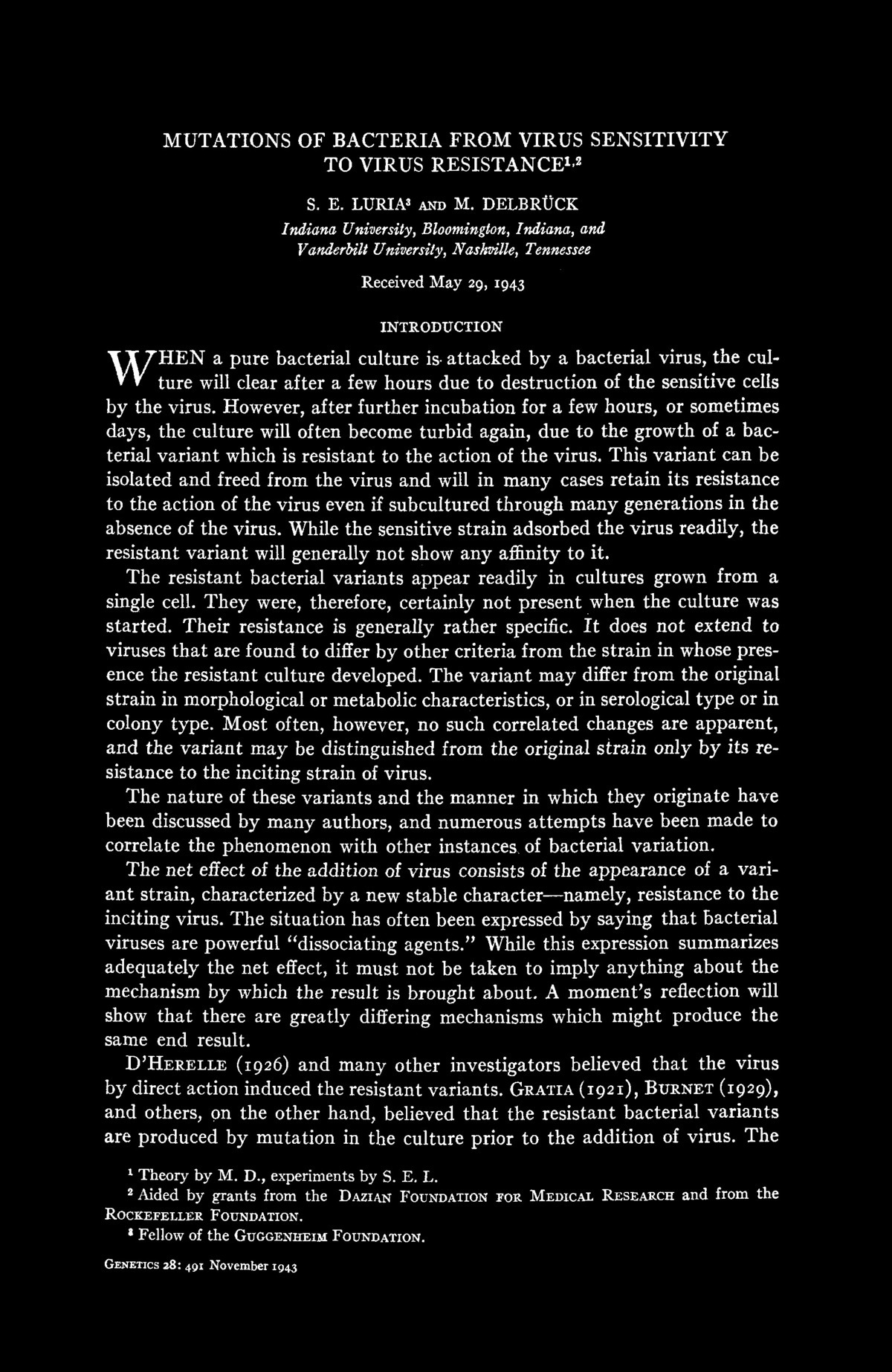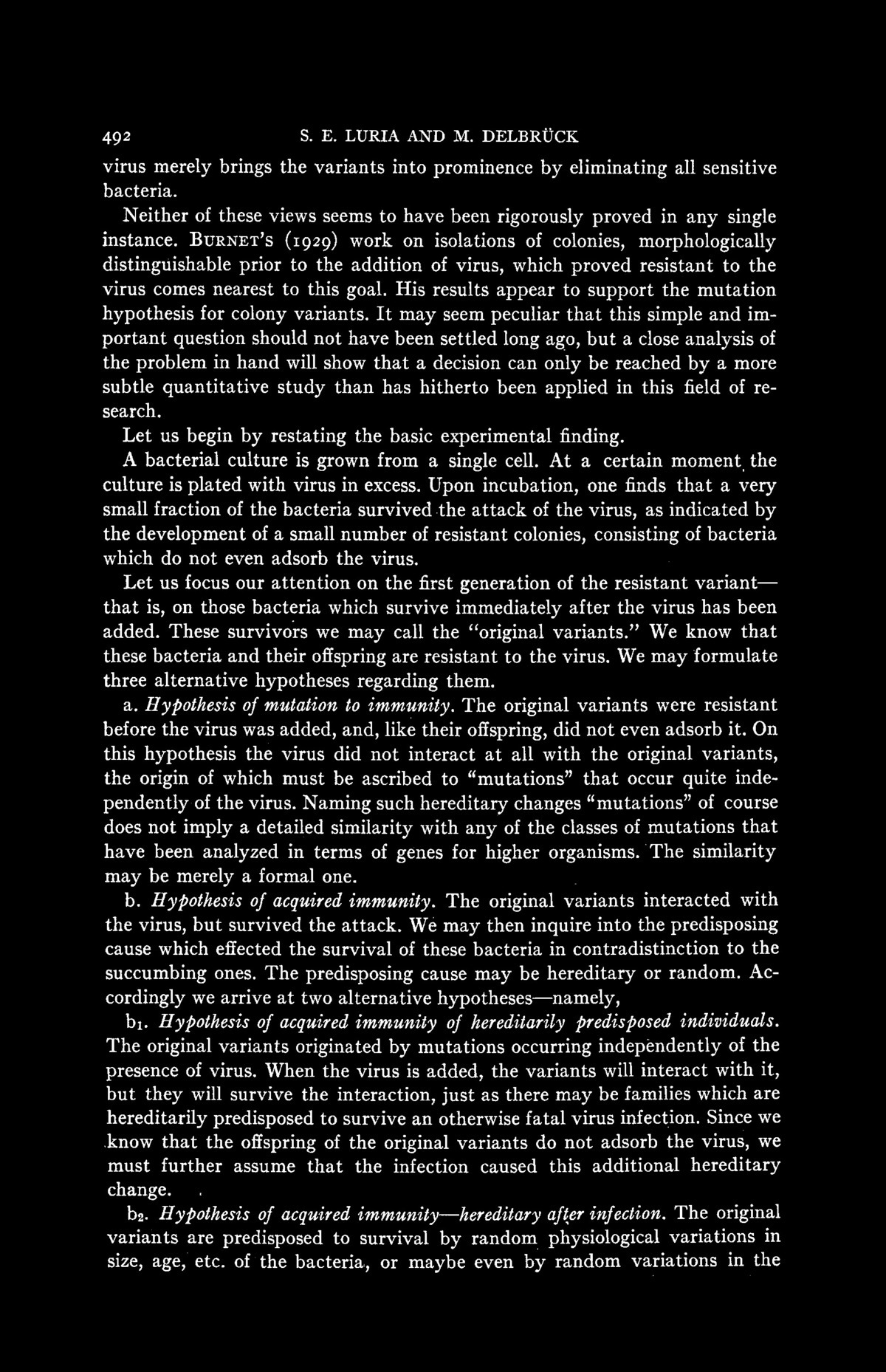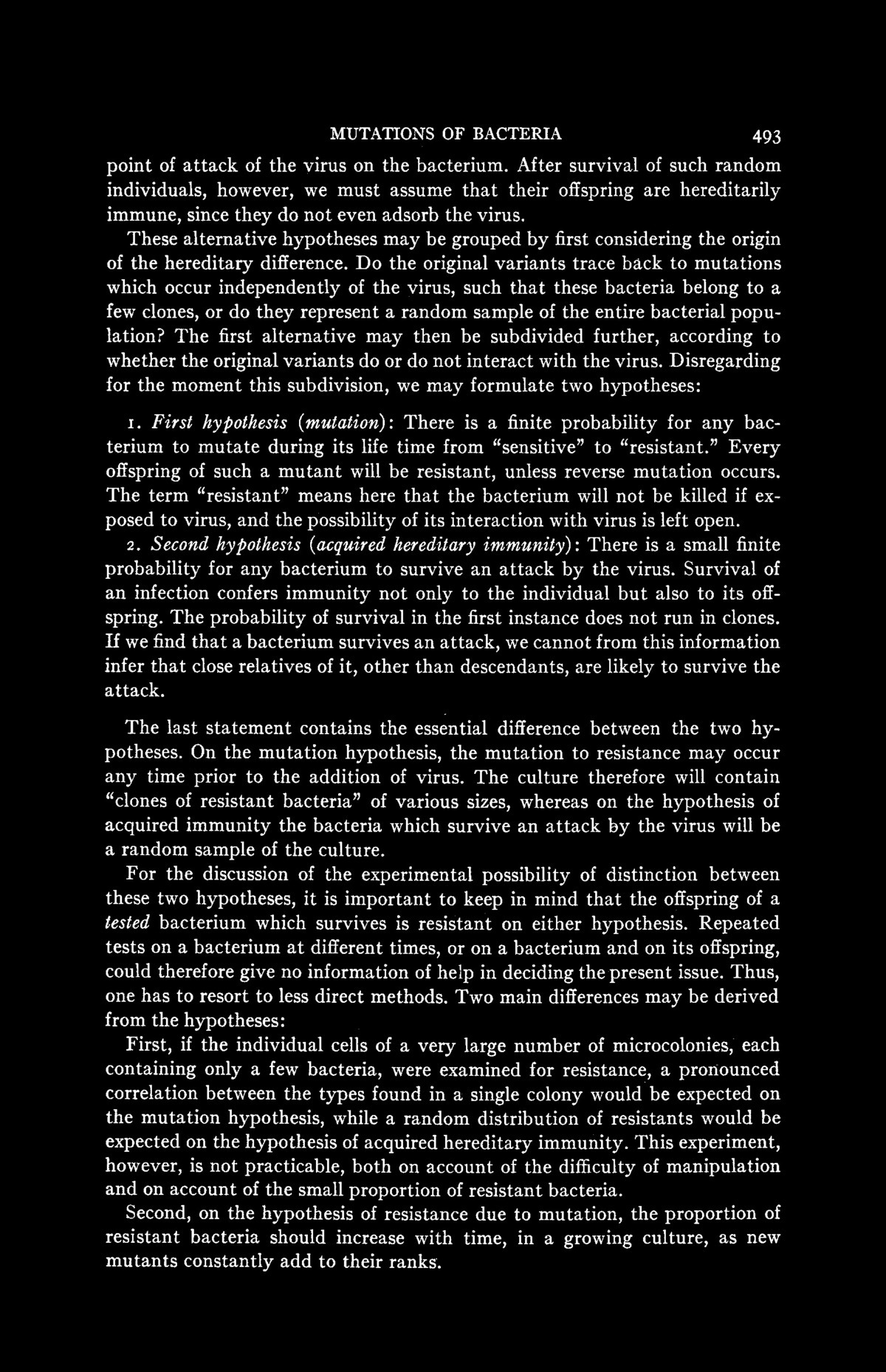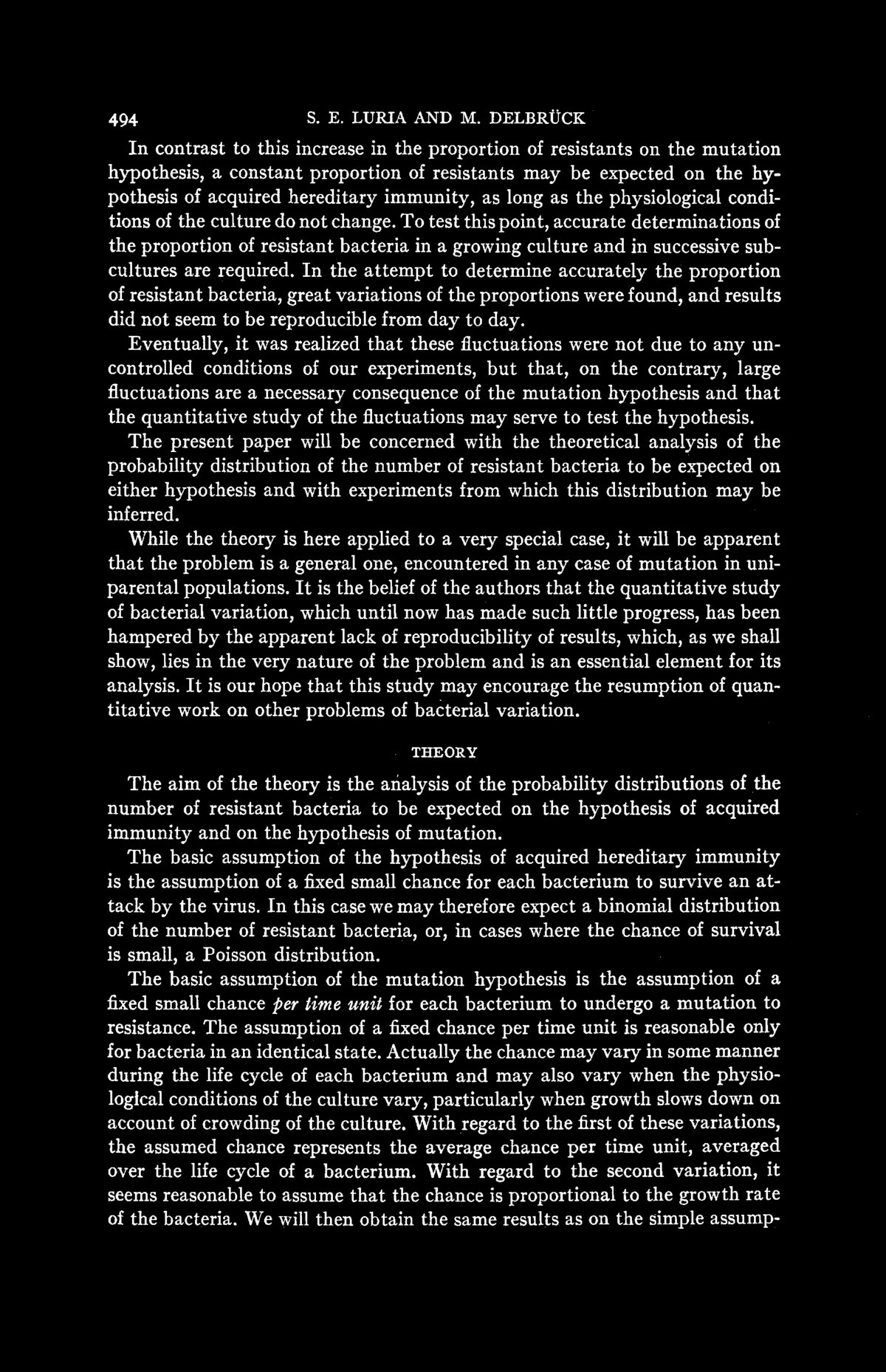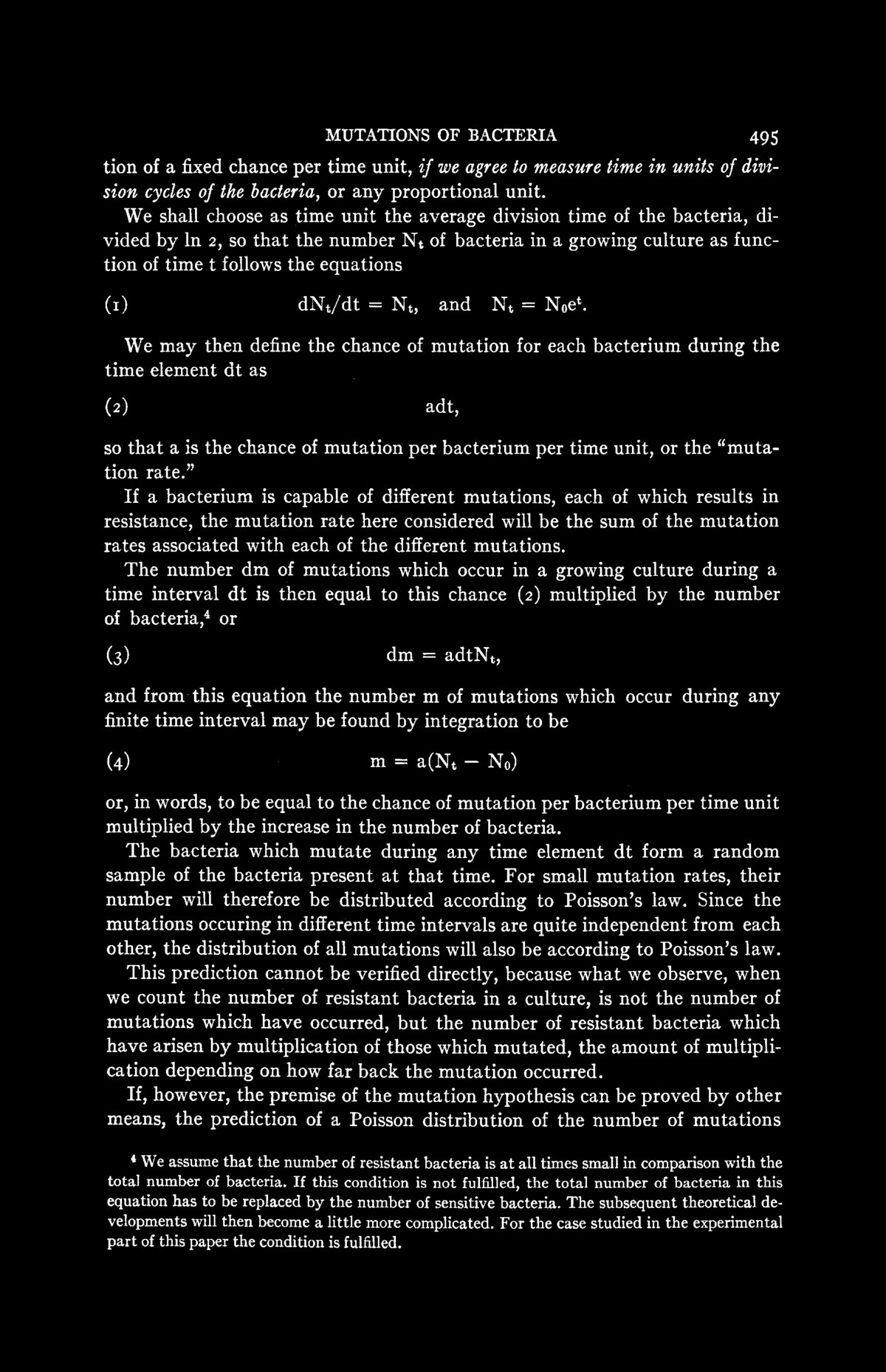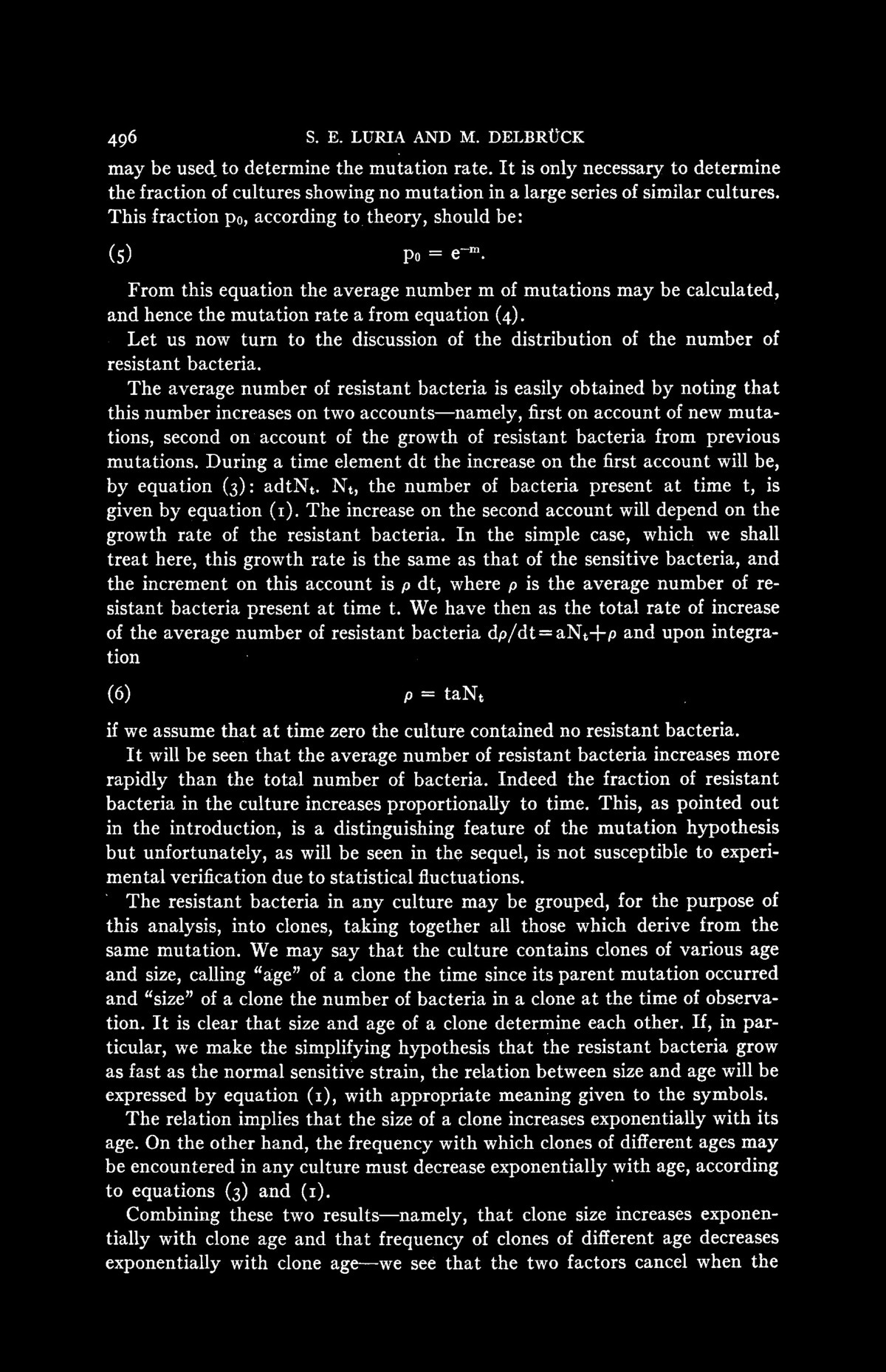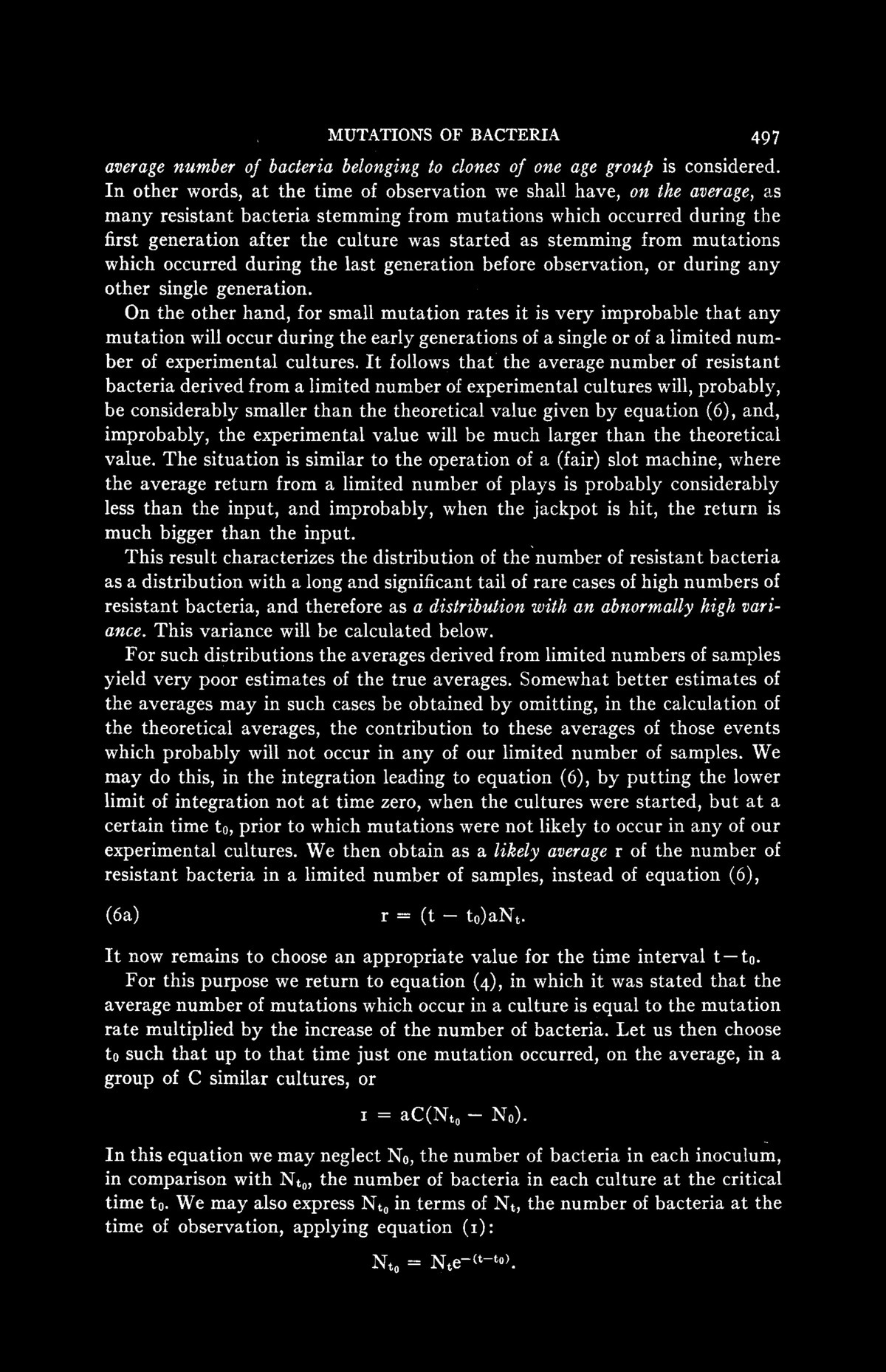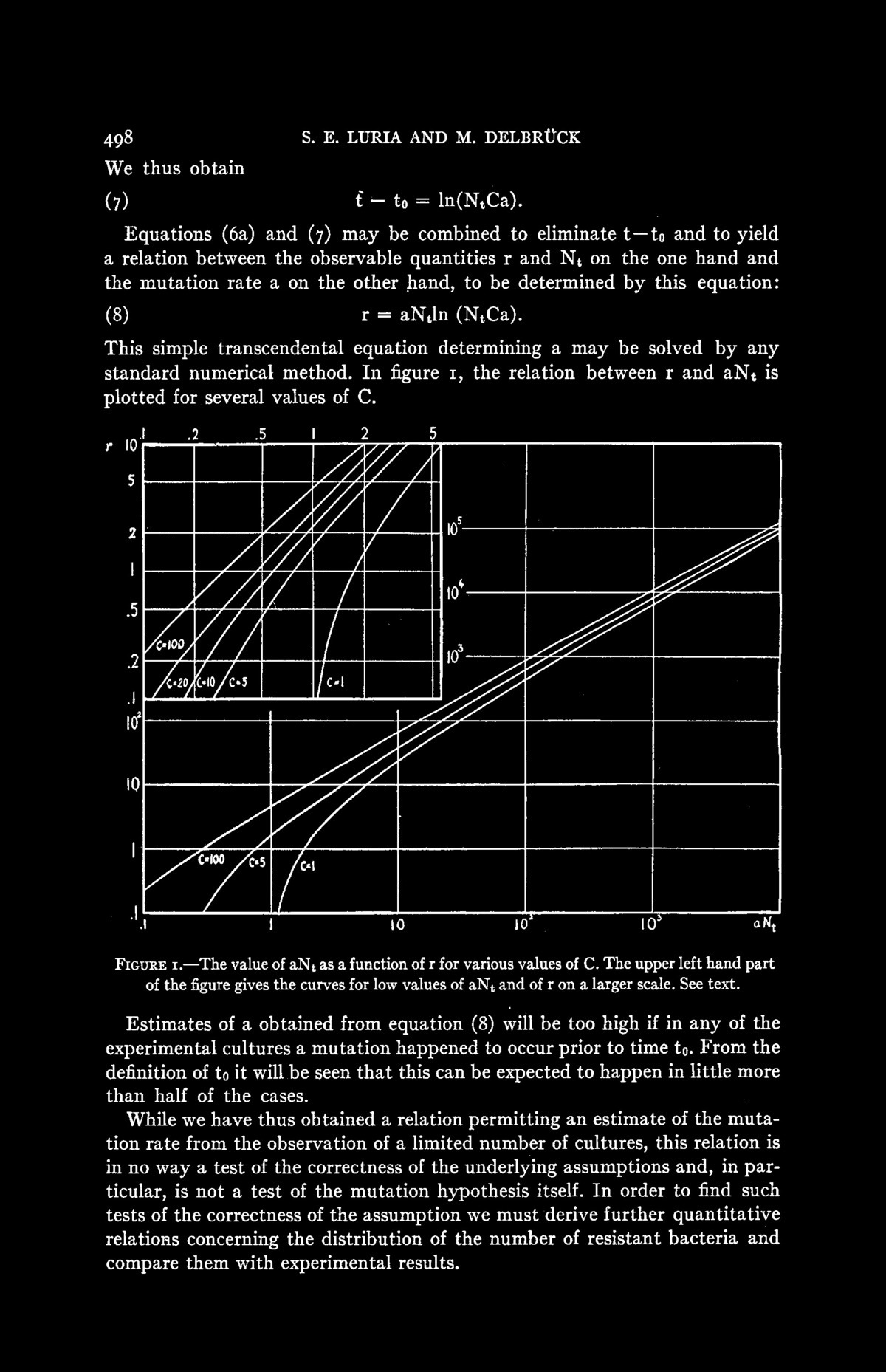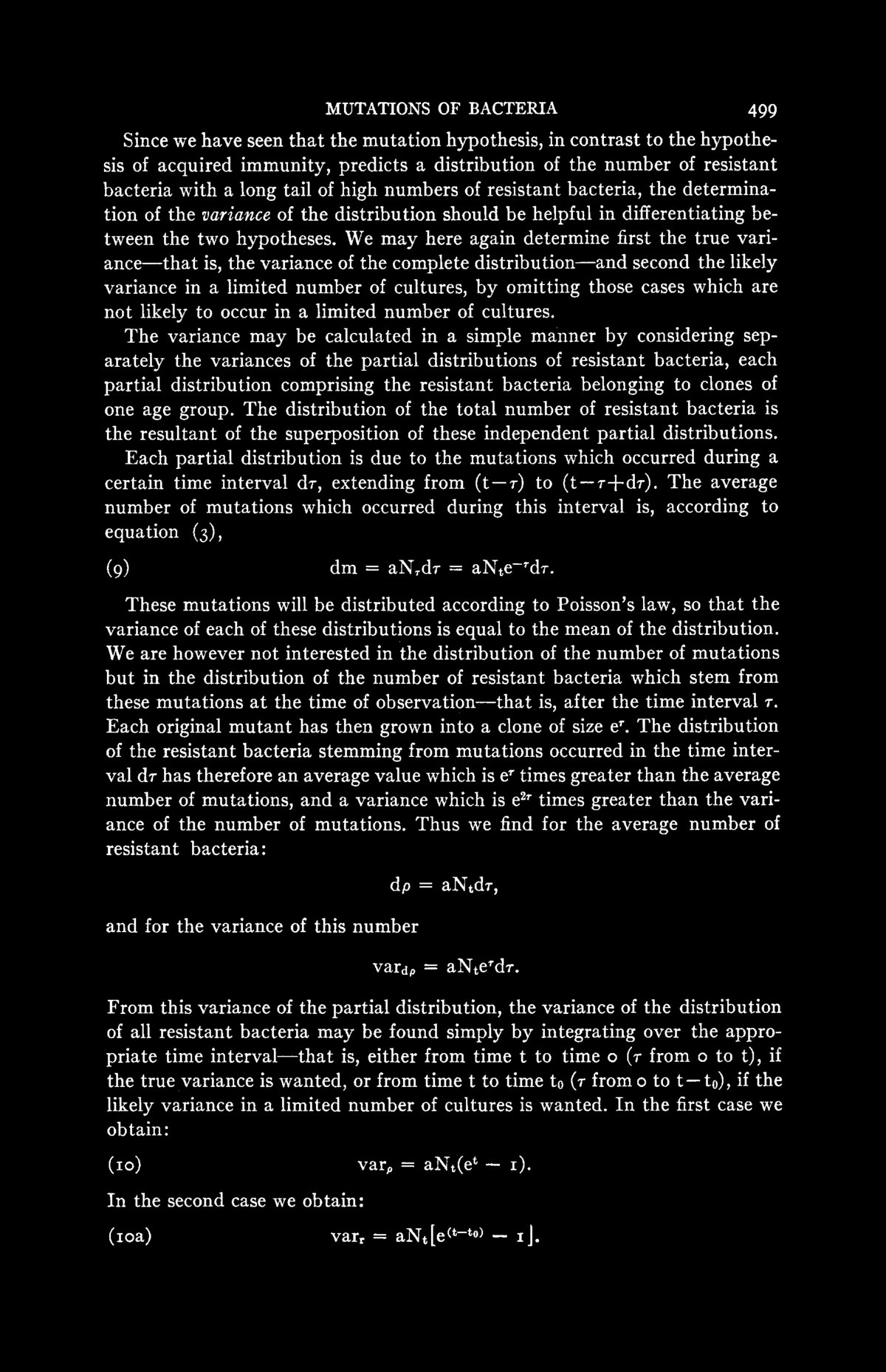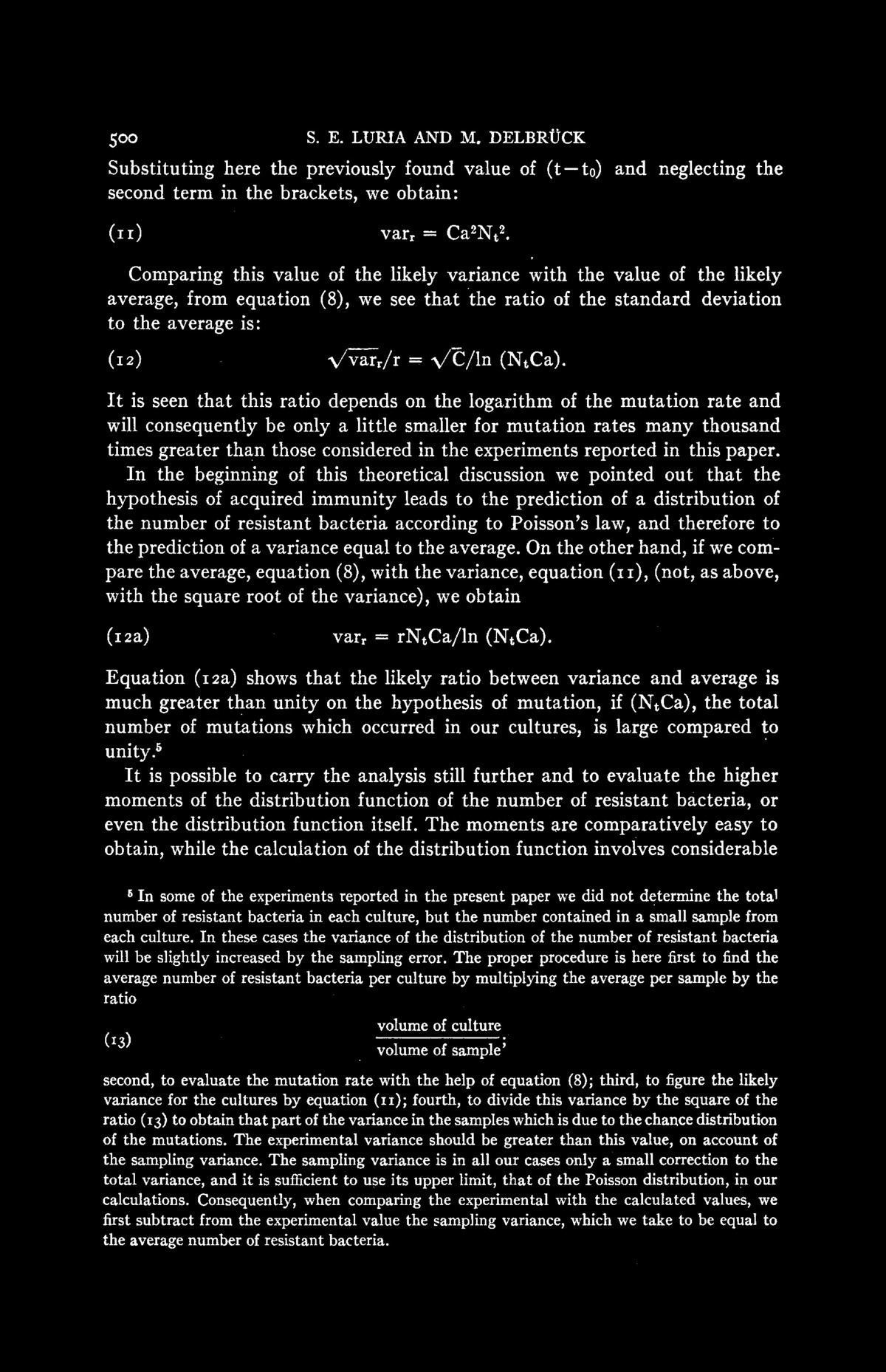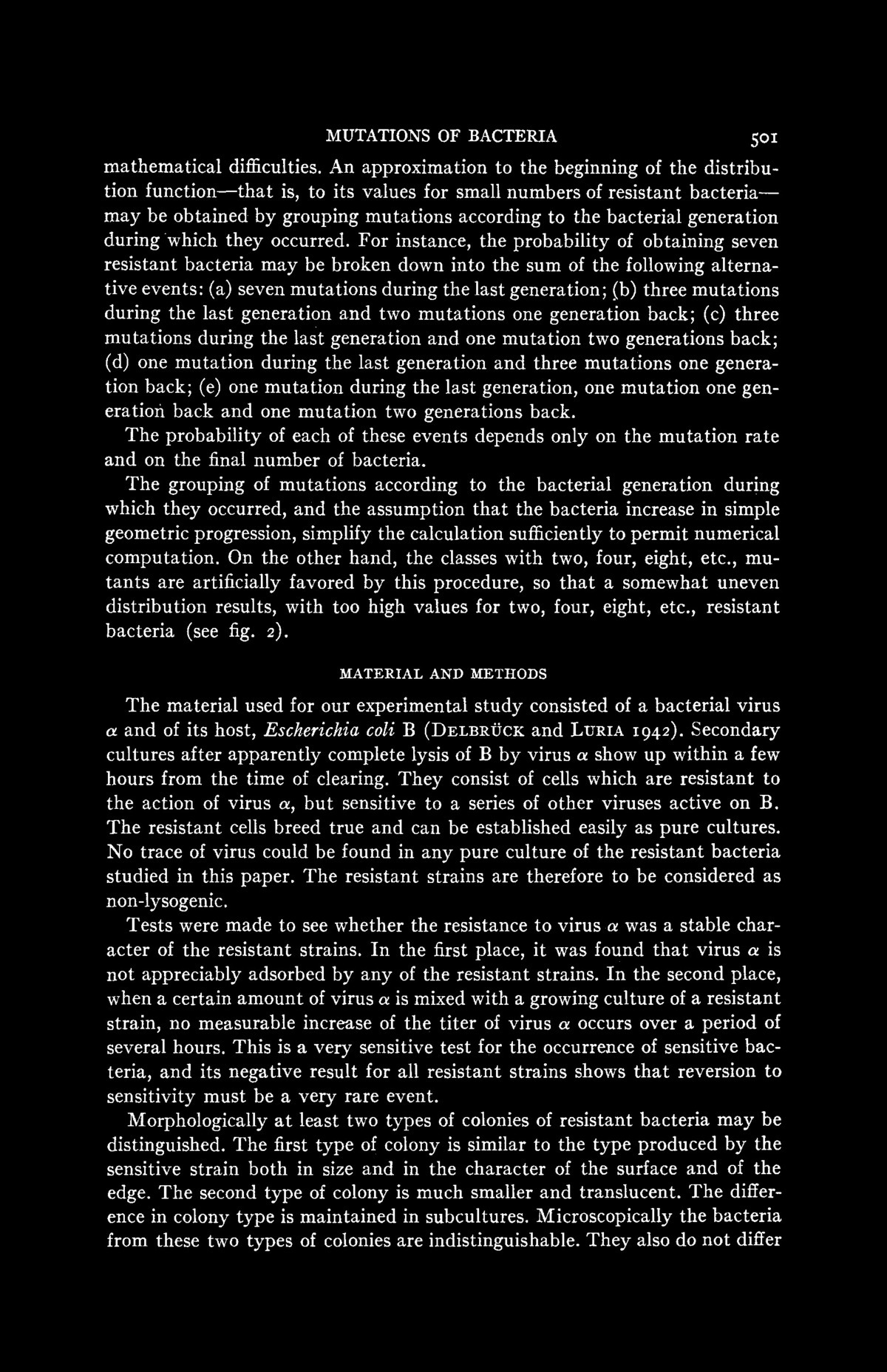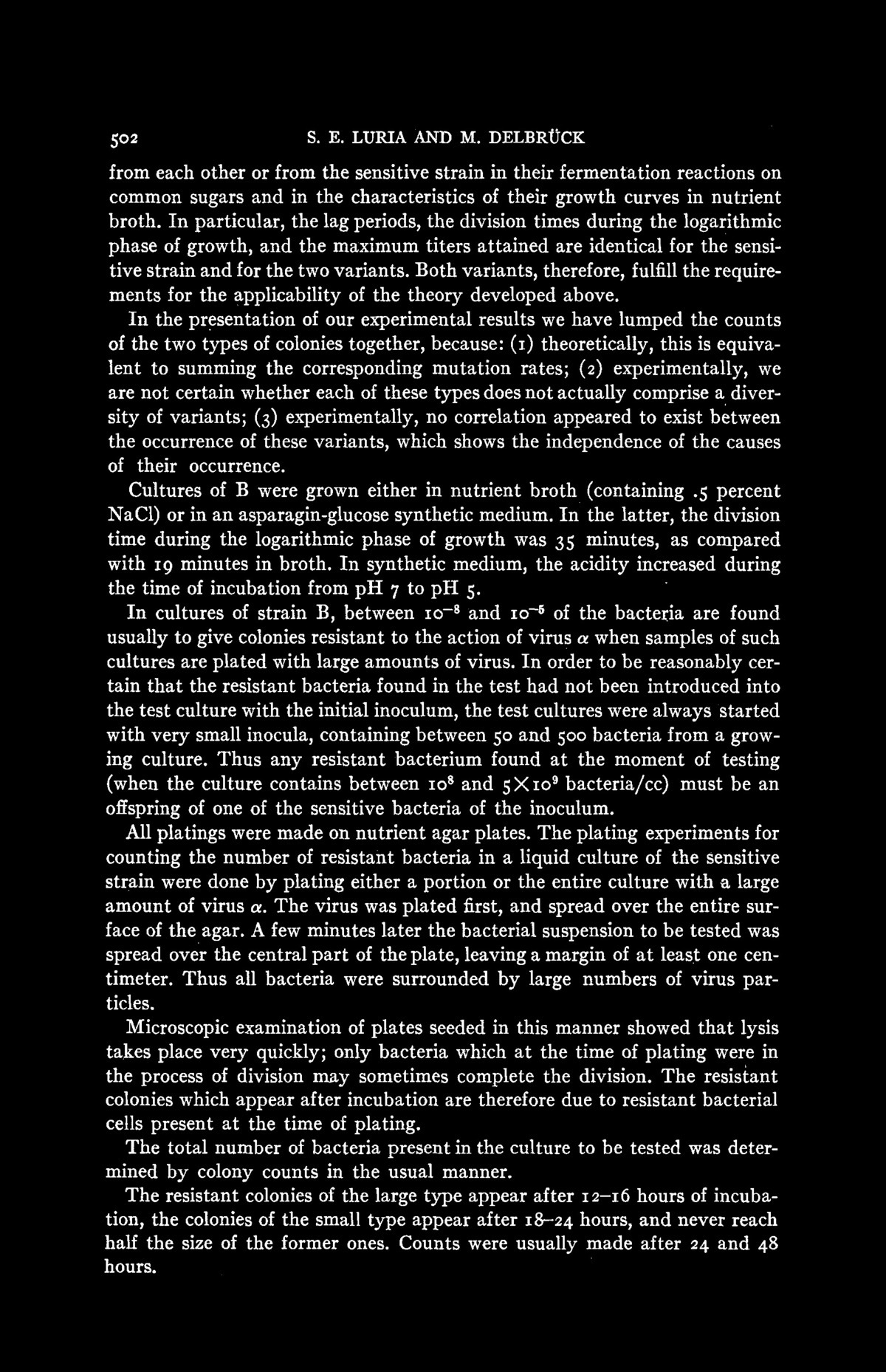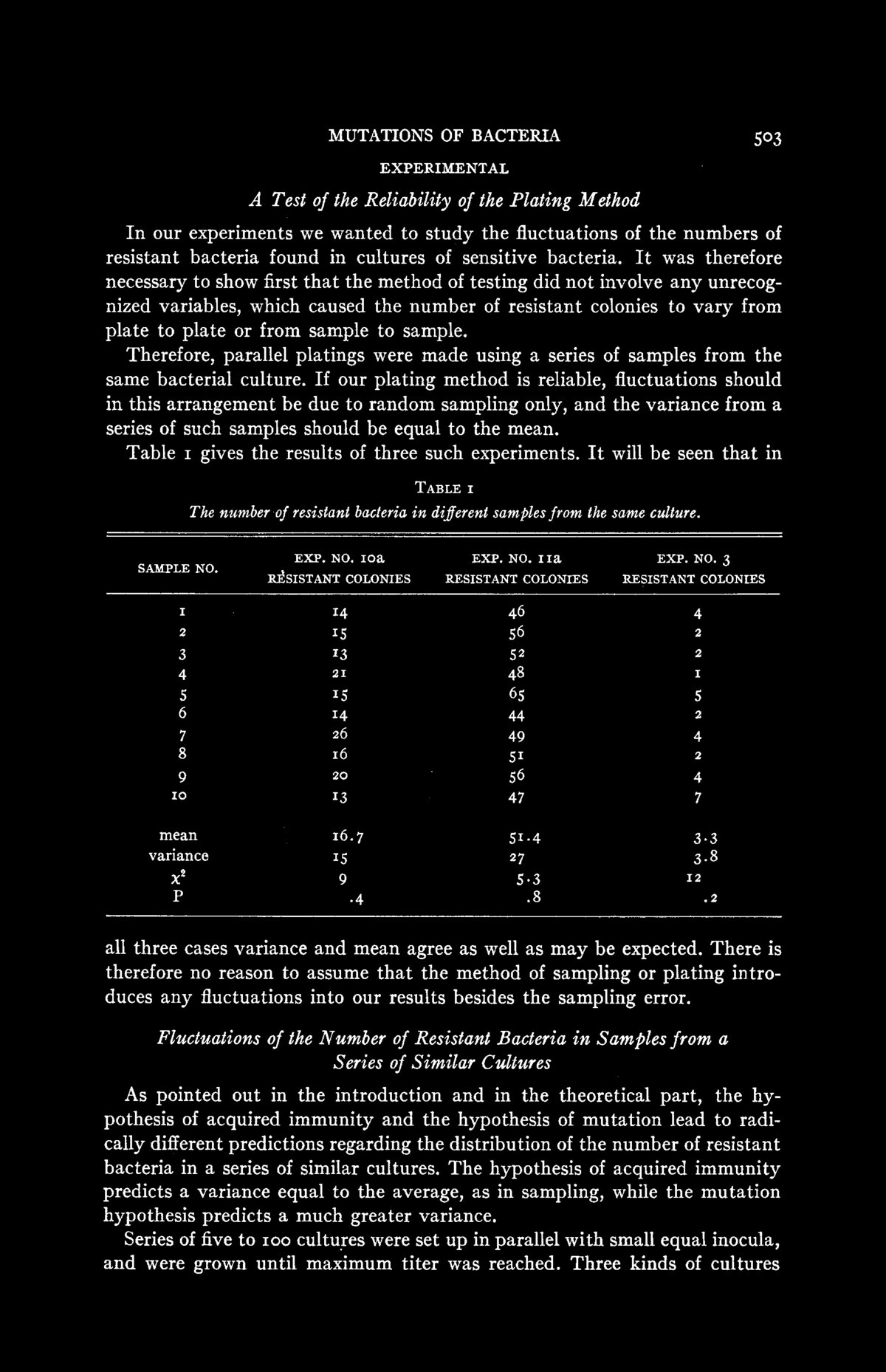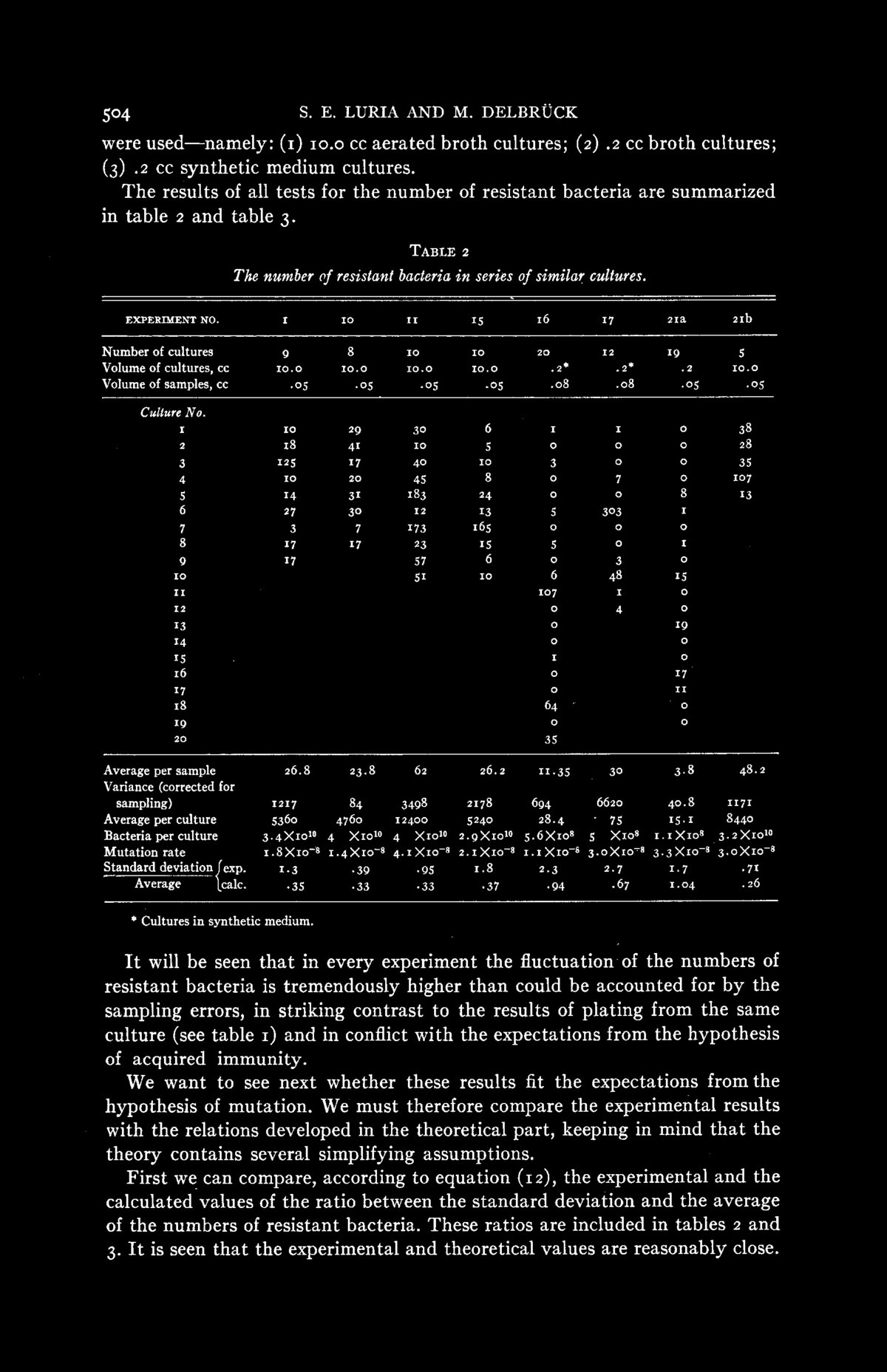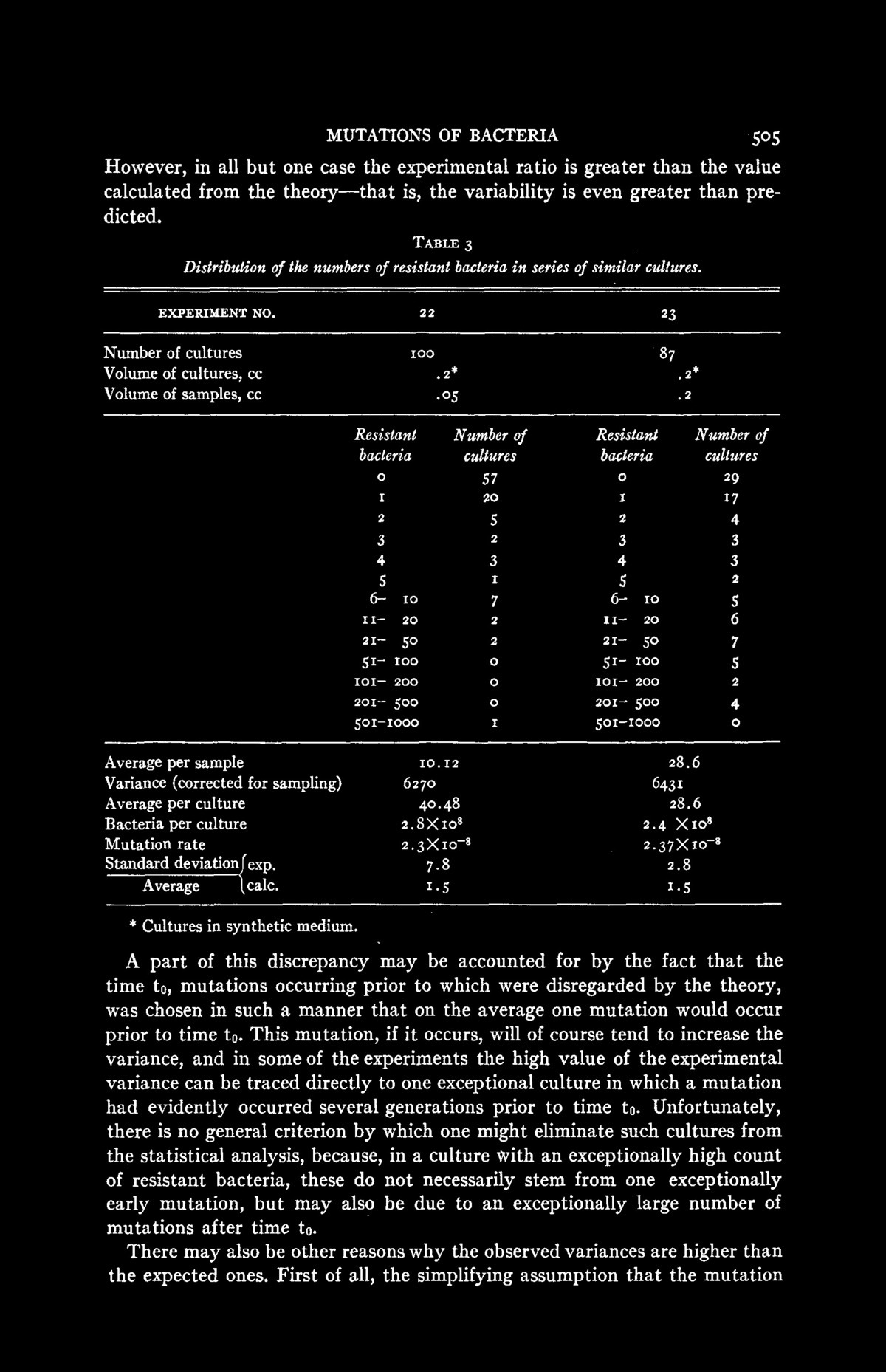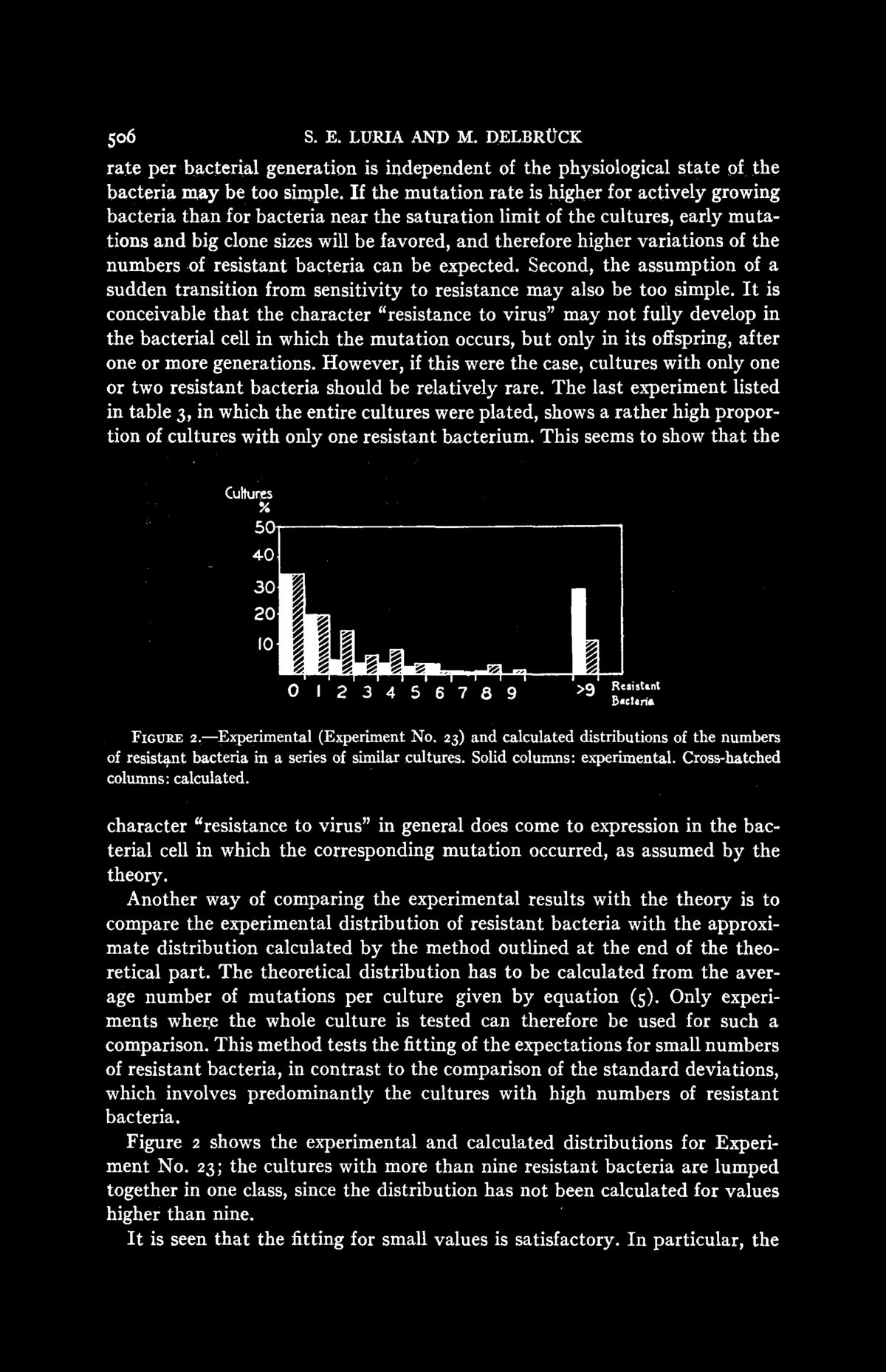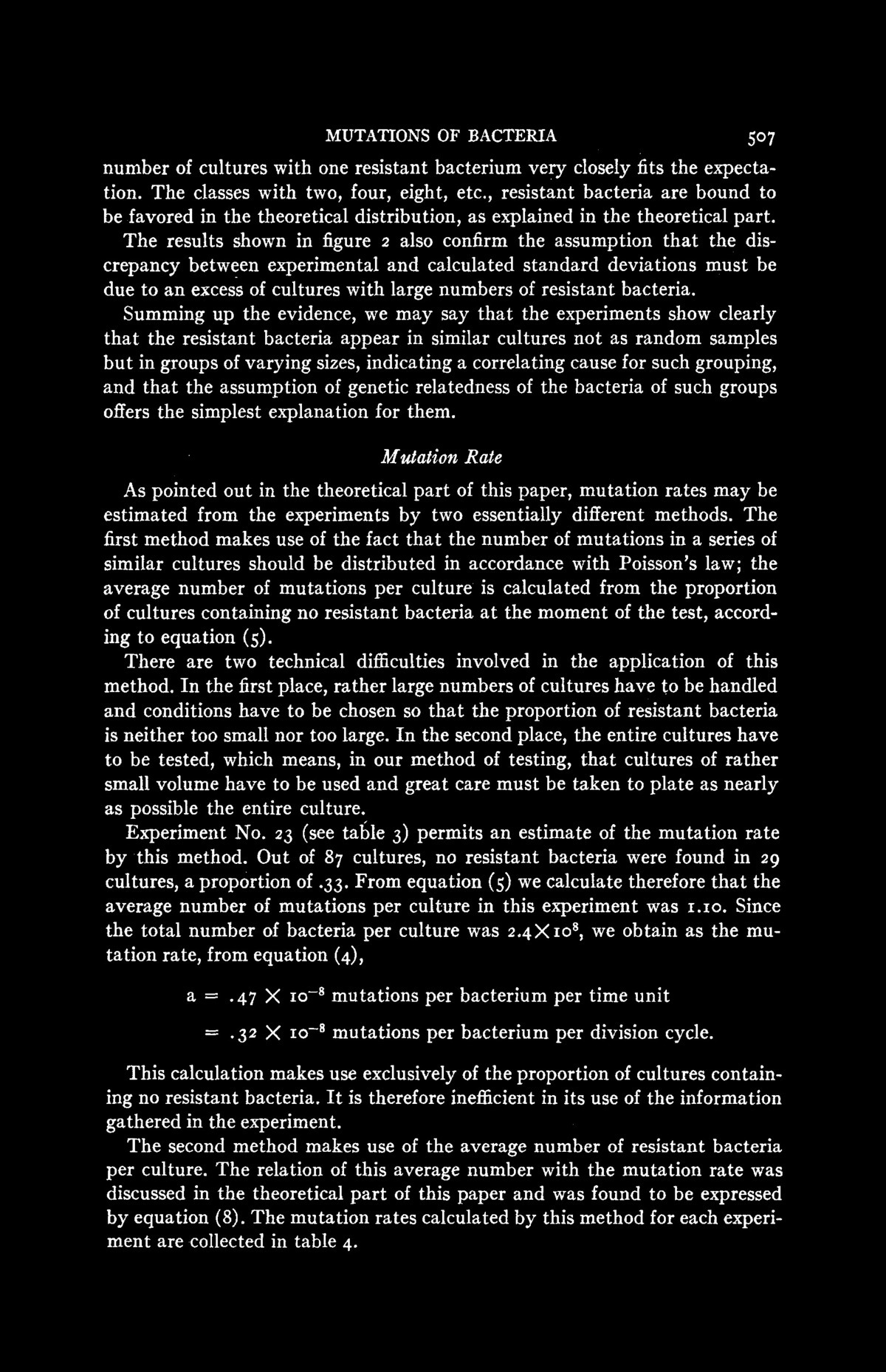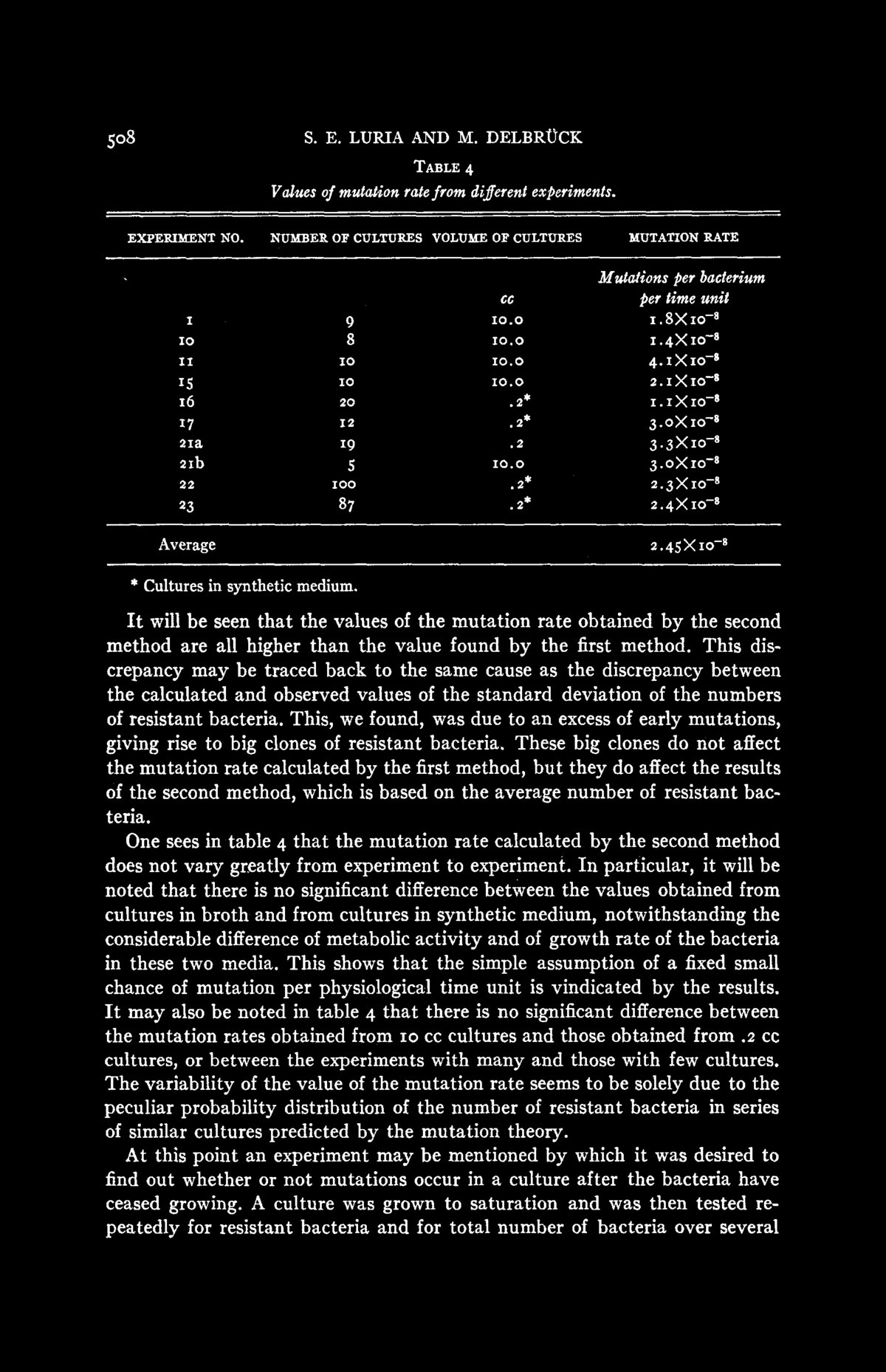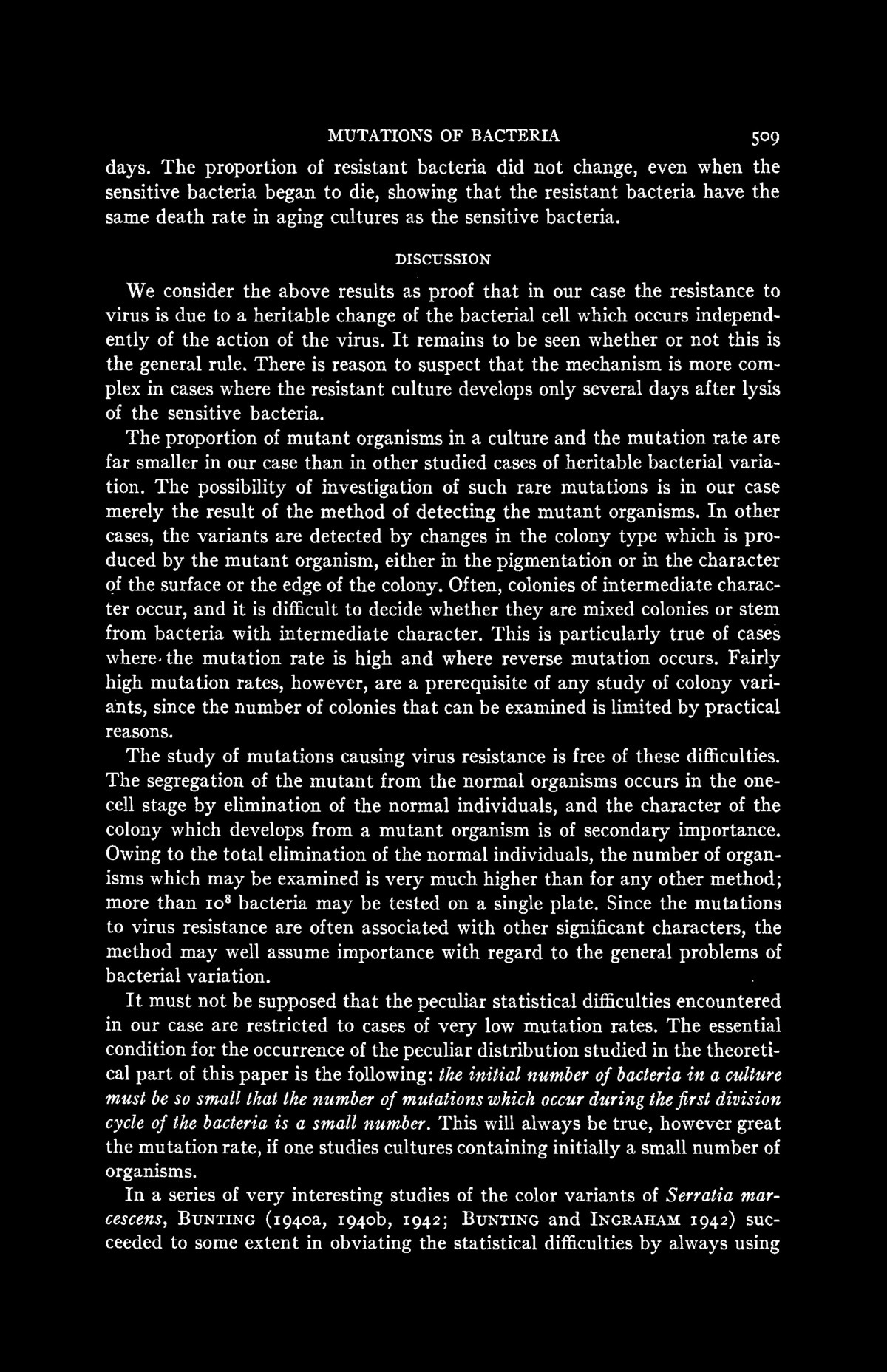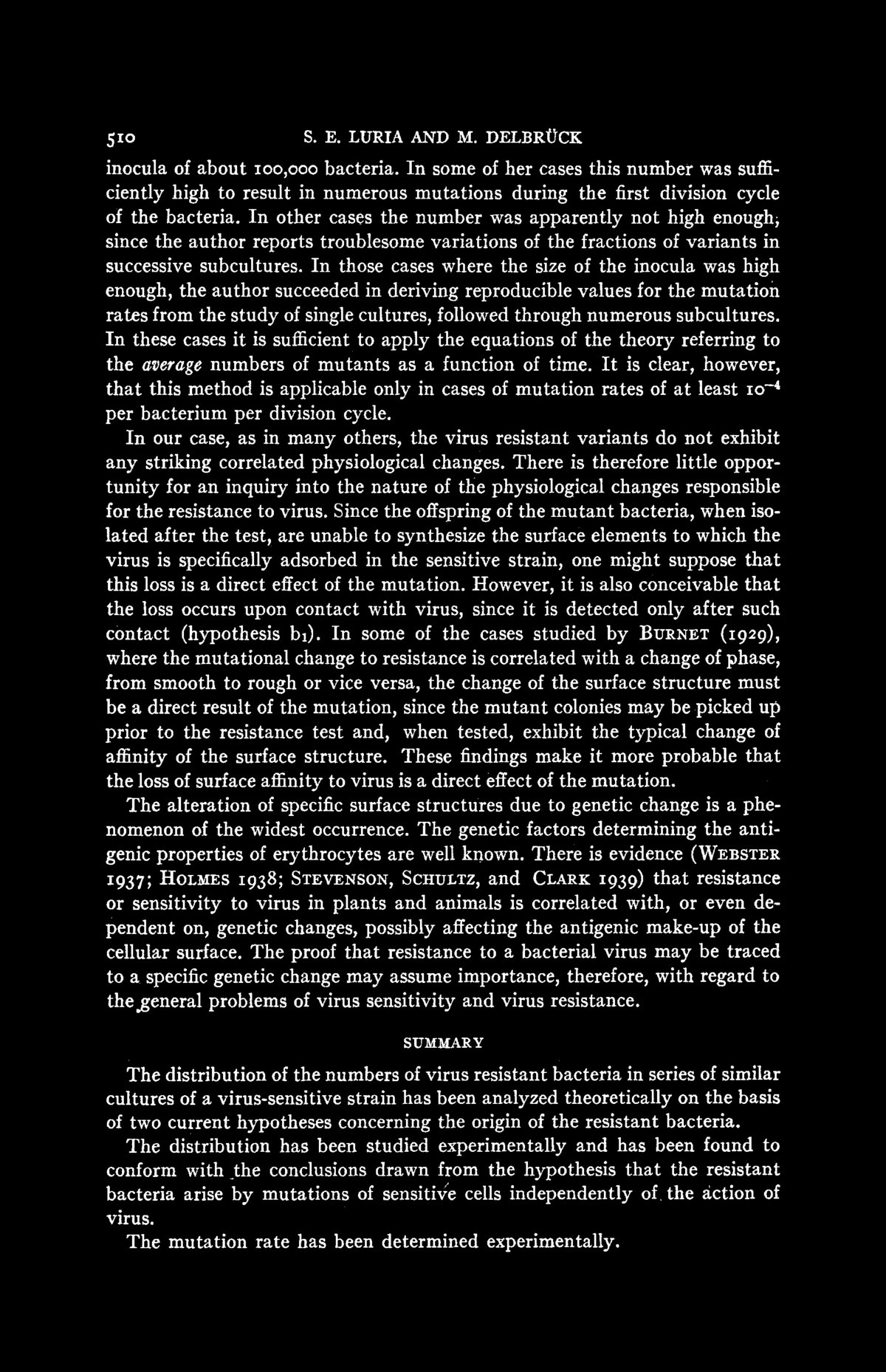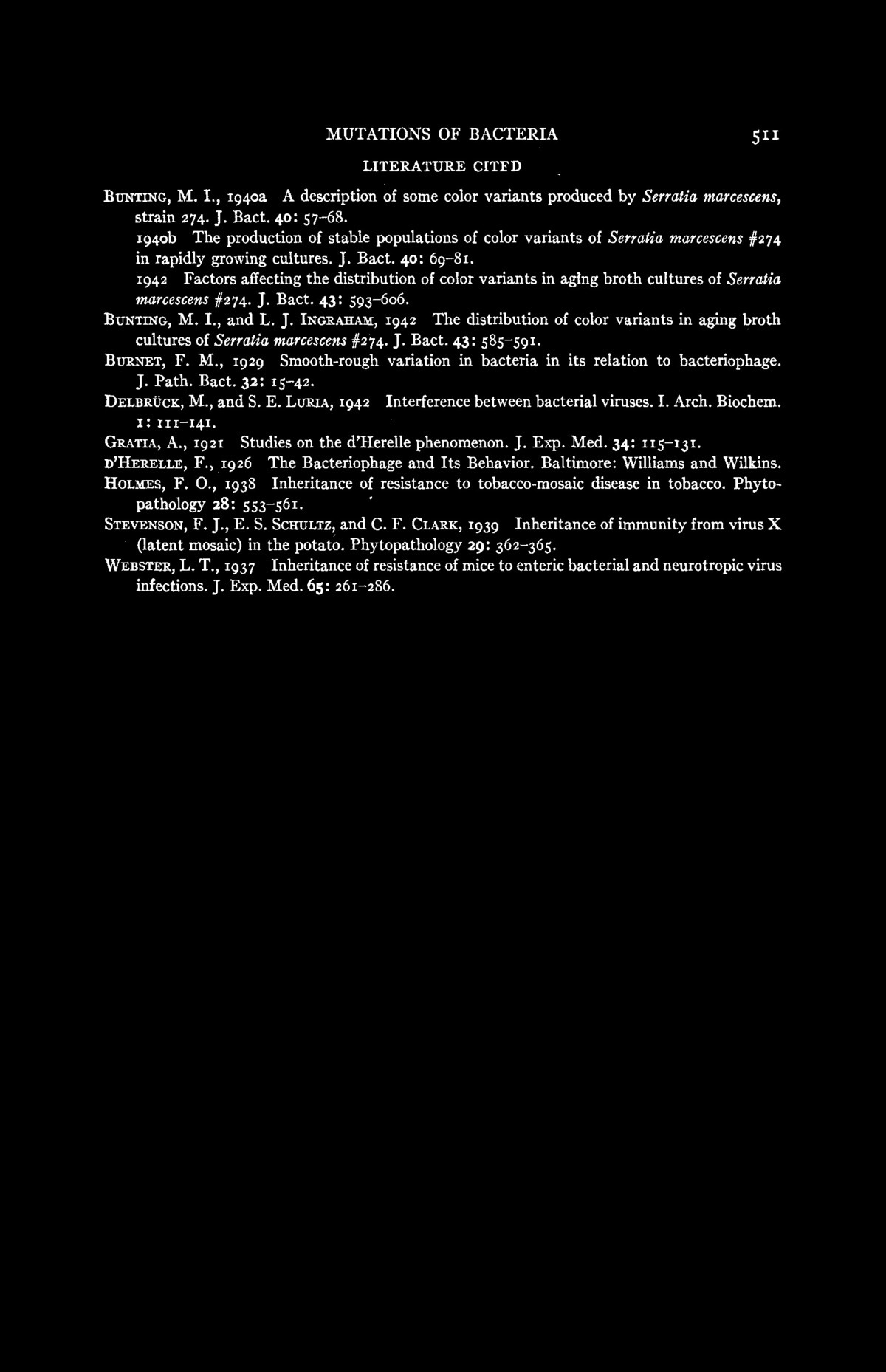# Darwin-Lamarck

Here are some illustrations to Chapter 11 in Cliff's notes which deals with the Luria-Delbrück Experiment an application of the binomial distribution. Its a nice application of statistics and illustrates how important the Binomial distribution is. Max Delbrück and Salvador Luria got the 1969 Nobel Prize in Physiology and Medicine for a 1943 experiment published in a paper "Mutations of Bacteria from Virus Sensitivity to Virus Resistance, Genetics 28 (1943), 491-511". Their experiment was in favor of Darwin's view. . The model deals with N bacteria, divided into K colonies. The bacteria are exposed to a phage, a virus attacking bacteria.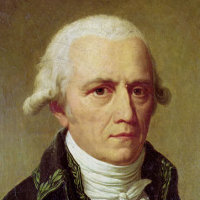Jean-Baptiste Lamarck, 1744-1829 Adaptive force: Selection force new traits to arise. Here is Lamarck's model. If the survival probability of a virus is p, then the probability that k bacteria survive is ```P[ X = n ] = (N!/(n!(N-n!)) pn (1-p)N-n ``` This random variable X has the expectation E[X] = N p and the variance Var[X] = N p(1-p) so that ```Var[X]/E[X] = 1-p ``` which is N independent and close to 1.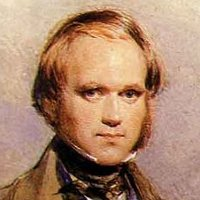Charles Darwin 1809-1882, Natural selection: Selection due to environmental stresses Darwin's proposal assumes there is a small probability q that one of the colonies has a mutation rendering it immune to the virus. Let Y be the random variable counting the number of colonies with this mutation. ```P[ Y = k ] = (K!/(k!(K-k!)) qk (1-q)K-k ``` The random variable Y has the expectation E[Y] = K q and variance Var[Y] = K q (1-q). But now, if k colonies have found a "cure" to the virus attack, we expect ``` P[X = n] = sumk=1K P[Y=k] k (N/K) ``` bacteria to survive. The random variable X has now expectation qN and variance q(1-q)N2, so that ```Var[X]/E[X] = (1-q) N ``` which is N dependent and grows exponentially in time.

## The article of Luria-Delbrück